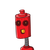### 1 thought on “Find a first digree polynomial with p(1)=1 and p(2) = 3 please help me by giving the correct answer it’s urgent​”

1.its 2x-1

Step-by-step explanation:

this is the first degree polynomial with p(1)=1 and

to calculate this answer you need to take a common first degree polynomial i.e. p(x)= ax + c

now, putting x= 1

we get, p(1)= a+c which is according to question equal to 1, i.e. a + c = 1

similarly, p(2) = 2a + c which is equal to 3

so, 2a + c = 3

we can write it as a + a + c = 3

i.e. a + 1 = 3 { from p(1) }

so, a= 2

putting it in any equation we get, c= -1

so the ans is, 2x-1